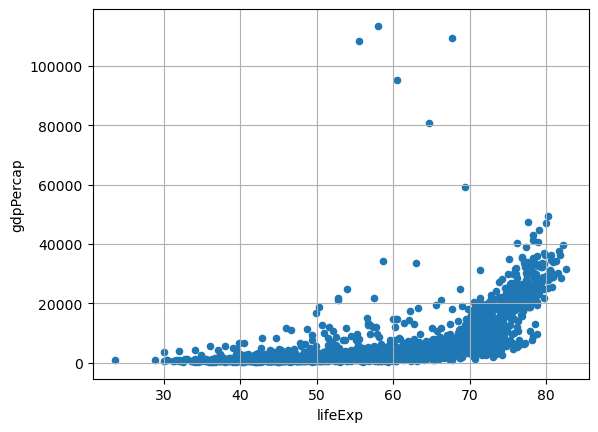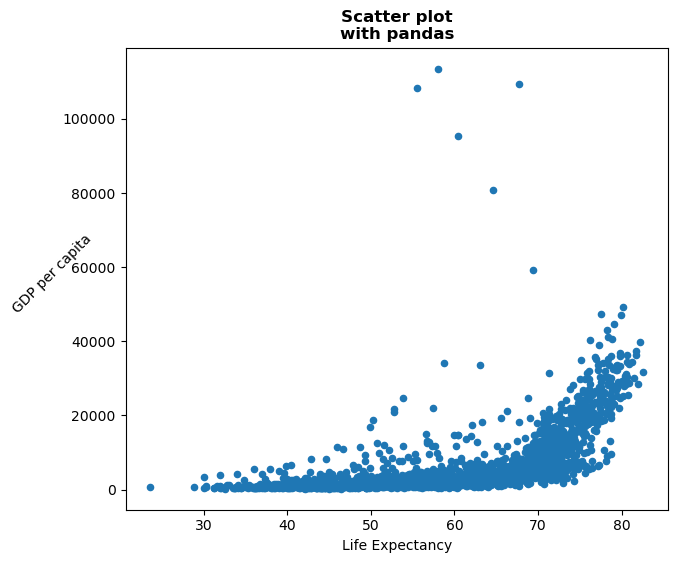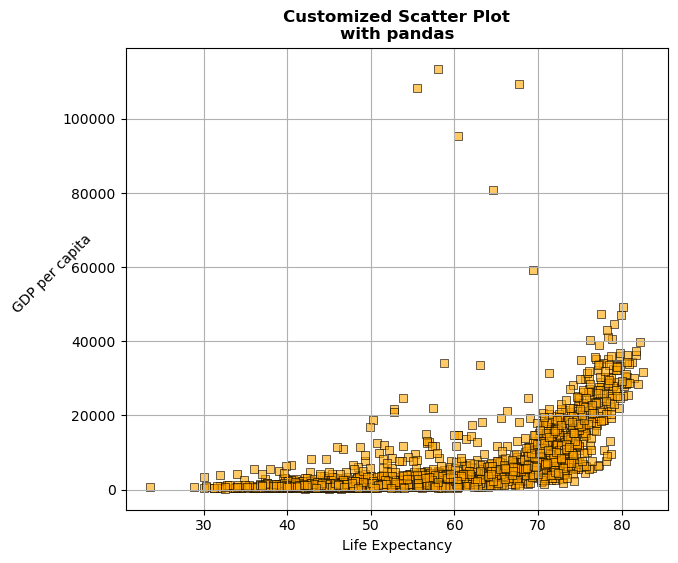A scatter plot is a graphical representation of data points in a dataset, where individual data points are plotted on a two-dimensional coordinate system. This type of plot allows us to visualize the relationship between two variables by showing how they are distributed across the plot.
Pandas, a powerful data manipulation library in Python, allow us to create easily scatter plots: check this introduction to scatter plots with pandas. In this post, we will explore how to leverage `Pandas` to customize scatter plots, making it good looking and studying available options.

## Libraries

Pandas is a popular open-source Python library used for data manipulation and analysis. It provides data structures and functions that make working with structured data, such as tabular data (like `Excel` spreadsheets or `SQL` tables), easy and intuitive.

To install Pandas, you can use the following command in your command-line interface (such as `Terminal` or `Command Prompt`):

`pip install pandas`

Matplotlib functionalities have been integrated into the pandas library, facilitating their use with `dataframes` and `series`. For this reason, you might also need to import the matplotlib library when building charts with Pandas.

This also means that they use the same functions, and if you already know Matplotlib, you'll have no trouble learning plots with Pandas.

``````import pandas as pd
import matplotlib.pyplot as plt``````

## Dataset

In order to create graphics with Pandas, we need to use pandas objects: `Dataframes` and `Series`. A dataframe can be seen as an `Excel` table, and a series as a `column` in that table. This means that we must systematically convert our data into a format used by pandas.

Since scatter plots need quantitative variables, we will get the Gap Minder dataset using the `read_csv()` function. The data can be accessed using the url below.

``````url = 'https://raw.githubusercontent.com/holtzy/The-Python-Graph-Gallery/master/static/data/gapminderData.csv'

## Basic scatter plot

Once we've opened our dataset, we'll now create the graph. The following displays the relation between the life expectancy and the gdp/capita using the `scatter()` function. This is probably one of the shortest ways to display a scatter plot in Python.

``````df.plot.scatter('lifeExp', # x-axis
'gdpPercap', # y-axis
grid=True, # Add a grid in the background
)
plt.show()``````## Format layout

Here we'll see how to remove the background grid, add some labels and the change the size of the figure. The main difference with the previous code chunk is that we save the object used to create the graph in `ax` and use it to add elements (like title) to the chart.

• remove grid: we just have to remove the `grid=True` argument
• add labels: `set_title()` and `set_xlabel()` functions
• change the figure size: add the `figsize(width,height)` argument when using the `scatter()` function
``````ax = df.plot.scatter('lifeExp', # x-axis
'gdpPercap', # y-axis
figsize=(7,6)
)

# Add title and labels ('\n' allow us to jump rows)
ax.set_title('Scatter plot\nwith pandas',
weight='bold')
ax.set_xlabel('Life Expectancy')
ax.set_ylabel('GDP per capita',
rotation=45) # rotate 45°

# Show the plot
plt.show()``````## Custom markers

The main component of a scatter plot is the markers, or the points. You can customize them a lot with Pandas. Here's what we'll do:

• type: `marker` argument (must be in the following list: `o`, `s`, `d`, `^`, `v`, `<`, `>`, `p`, `*`, `+`, `x`, `h`, `1`, `2`, `3`, `4`)
• color: `color` argument
• color of the edges: `edgecolor` argument
• size: `s` argument
• opacity: `alpha` argument
• width of the edge: `linewidth` argument
``````# Create the scatter plot with custom markers
ax = df.plot.scatter('lifeExp', # x-axis
'gdpPercap', # y-axis
figsize=(7,6),
marker=',',  # Use circles as markers
color='orange',  # Marker color
edgecolor='black',  # Marker edge color
s=40,  # Marker size
alpha=0.6,  # Marker transparency
linewidth=0.7, # Width of the edges
grid=True
)

# Add title and labels ('\n' allows us to jump rows)
ax.set_title('Customized Scatter Plot\nwith pandas', weight='bold')
ax.set_xlabel('Life Expectancy')
ax.set_ylabel('GDP per capita', rotation=45)

# Show the plot
plt.show()``````## Going further

This post explains how to customize title, axis and markers of a scatter plot built with pandas.

For more examples of how to create or customize your plots with Pandas, see the pandas section. You may also be interested in how to customize your scatter plots with Matplotlib and Seaborn.

## Contact & Edit

👋 This document is a work by Yan Holtz. You can contribute on github, send me a feedback on twitter or subscribe to the newsletter to know when new examples are published! 🔥